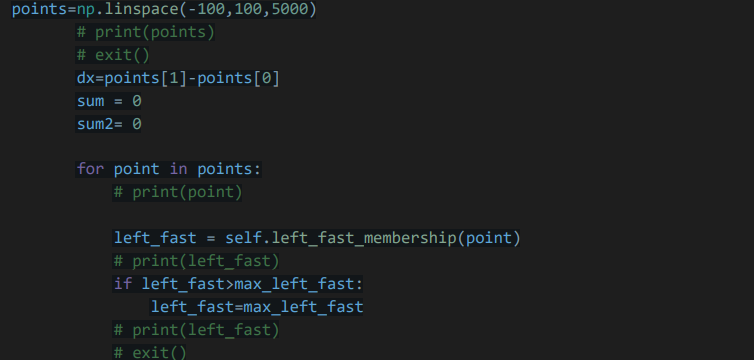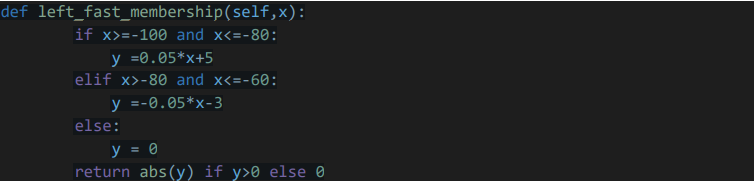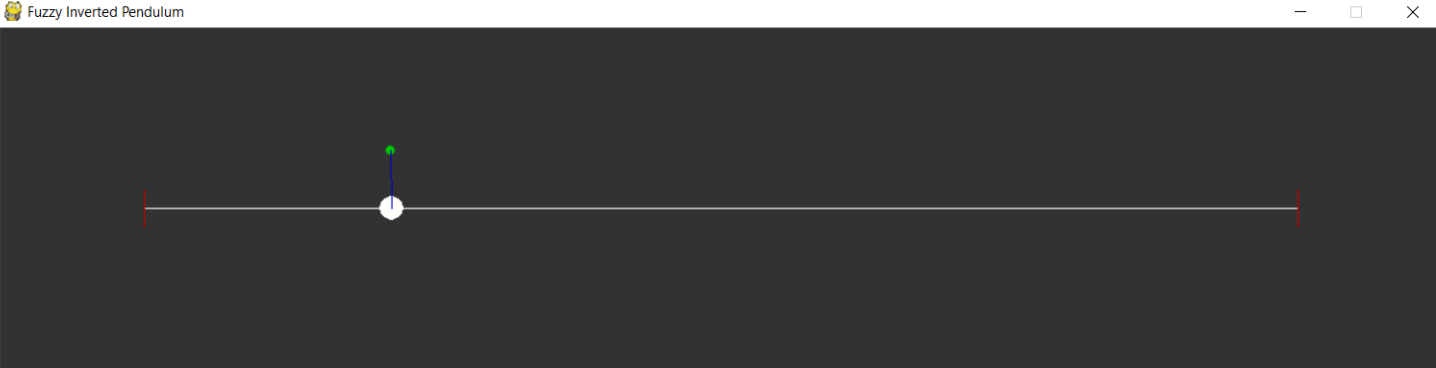inverted-pendulum-fuzzy-control-

inverted pendulum fuzzy control python code (python 2.7.18)
implemented controller.py

We have 3 general functions for 3 main steps:
fuzzification function 1
In this function the input is received and given by the given figures and the calculation of the line equation
We each calculate the function of their belonging to the fuzzy set.
pa: REAL; (* description = ‘pendulum angle’, min = 0, max = 360,
unit = ‘degrees’ )
pv: REAL; (
description = ‘pendulum angular velocity’, min = –
200, max = 200, unit = ‘degrees per second’ *)
The output of this function is 15 functions belonging to pa and pv.
[up_more_right, up_right, up, up_left, up_more_left, down_more_left, do
wn_left, down, down_right, down_more_right]
[cw_fast_pv, cw_slow_pv, stop_pv, ccw_slow_pv, ccw_fast_pv]
inference function 2
In this function, 15 outputs of the previous function are given as input.
Using the 43 rules mentioned 5 lists of the output belonging function of these 43 rules as
power We set the fuzzy belonging set to force and give it a maximum of 5 lists of belonging functions
Return the output title.
function 3
Using the 5 inputs of the center of mass function of the shape resulting from the collision of this maximum
We get the inputs and the fuzzy force set by the integration method and get it
We return in the form of output force.
Example To check a membership to a fuzzy force set:Finally, our output is as follows:View Github